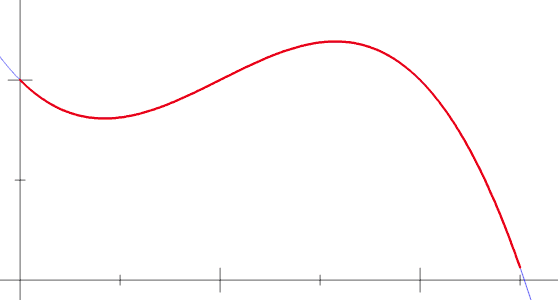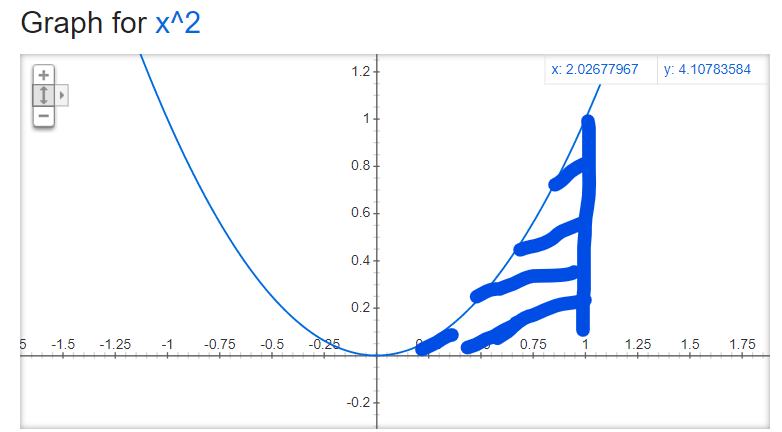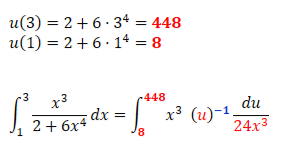top of page
Search

# Integrals and integration tutorialKey concepts: Integrals/integration Definite and indefinite integrals Substitution Let's face it: Doing integrals and doing them right is pretty hard. I remember learning about them for the first time and having no clue what they meant or how to get started solving my homework. Let us walk through some example problems and see how integration actually works! Question 1: "Draw a graph of the function x^2 and determine the area between the curve and the x-axis on the interval from 0 to 1." Alright, let us give it a shot. Below, I have graphed the function and crudely sketched out the area we are interested in.How big is that area? It is not shaped like a square, a circle or a triangle, so we can't just break out a ruler and measure it. Luckily, we can use definite integrals to calculate it! Let us write down the following:Basically we have to imagine chopping the area under the function up into a bunch of rectangles. They have a height x^2 and a width dx. Obviously this is not completely correct, because the rectangles will always be a bit too large. But if we make dx really small, then the extra area will also get really small.Calculating the "definite integral" of x^2 from 0 to 1 basically tells us what the area will be if we make dx infinitely small. What about "indefinite integrals"? Question 2 "Find the anti-derivative, F(x), of f(x)=x^3 so that F(4)=63" Hmm, that looks cryptic to say the least. What are we supposed to do here? For good measure, let us draw a graph of f(x):In Question 1, we were explicitly told that we should integrate from 0 to 1. We were looking for a specific area. Right now, we are being asked to find a general "area function, F" that tells us how area under f(x)=x^3 changes for different values of x. Also, we want to make sure that the area is 63 if x=4. Let us try to write down the "indefinite integral":The expression in the parenthesis basically tells us how the area under f(x) changes as x is changed. The weird +C tells us what the area should be for a particular value of x. To solve this problem correctly, we need to ensure that F(4)=63. Let us plug this information in to the expression:Now we can solve for C:Therefore the solution to the whole problem is:Question 3 "Find the area under the curve offrom 1 to 3" Let us graph the function for good measure:Look, I get what you are thinking: "Seriously?! I just figured out how to integrate basic stuff like x^5 and exp(x). That expression looks completely impossible to integrate. I mean it's a fraction of two different powers of x, for crying out loud! There is no way I can figure that out!" Am I a mind reader or what? Anyways, there is actually a super easy way to solve problems like this. It involves doing "integration by substitution". Here is the idea:We notice that the integral consists of some inner function stuffed inside an outer one. We then define:This allows us to rewrite the integral as:Now our goal is the following: Find a way to replace every x in the expression above with something that depends only on u Let us start by getting rid of dx. We do this by taking the derivative of u:Now we are going to use the best kind of trick in the world; a trick that both works AND infuriates pure mathematicians! We are going to solve for dx in the expression above, even though du/dx is not technically speaking a fraction:Now we are going to plug this back in to the integral:We are also going to change the integration bounds, 1 and 3, to:Notice how the expression now contains x^3/x^3=1. We can therefore simplify the expression:Now we just have to integrate 1/something. It turns out that the antiderivative of 1/u is:NICE! Let us just recap what we did:

1. We noticed that the expression we wanted to integrate had an outer function(inner function).

2. We defined u=inner function

3. We found du/dx=(inner function)'

4. We solved for dx and plugged it back in to the integral

5. We replaced the bounds x=a and x=b with u(a) and u(b)

6. We got lucky and saw that annoying, complicated factors cancelled out.

7. We got a much simpler integral out of it that we could easily solve.

Ok, so obviously I picked this particular f(x) because I knew in advance that x^3 would be cancelled out eventually. Integration by substitution is not some magical method that works for every single integral you come across. However, if you encounter an integral that contains some function inside another one, there is an very good chance that substitution will work! Try it out for